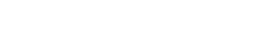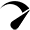# How to Calculate HPLC Column VolumeHow to Calculate HPLC Column Volume

High-Performance Liquid Chromatography (HPLC) is a widely used analytical technique for separating and analyzing complex mixtures. The column is the most critical component in the HPLC system, and its volume plays a crucial role in determining the separation efficiency and resolution of the analysis. Accurate knowledge of the column volume is essential for optimal method development and efficient use of the mobile phase. In this blog, we will discuss the process of calculating the HPLC column volume, including the determination of the internal diameter of the column, the calculation of the column volume, and the verification of the calculated volume.

Definition of HPLC Column Volume

The HPLC column volume refers to the total internal volume of the column, including the volume occupied by the stationary and mobile phases. The column volume is crucial in determining the amount of sample that can be injected and the residence time of the sample in the column. A larger column volume leads to a longer residence time and better separation but also requires a higher mobile phase flow rate to maintain the desired pressure and a higher sample size to achieve good sensitivity. On the other hand, a smaller column volume results in a shorter residence time, lower mobile phase flow rate, and improved sensitivity but can compromise the separation efficiency and resolution.

Importance of Knowing the Column Volume

Knowing the column volume is essential for several reasons, including:

• Optimal method development: Accurate knowledge of the column volume allows calculating the optimal mobile phase flow rate, which is crucial for achieving the desired separation efficiency and resolution.
• Efficient use of the mobile phase: A smaller column volume leads to a lower mobile phase flow rate, which conserves the mobile phase and reduces the analysis cost.
• Injection volume optimization: Accurate knowledge of the column volume allows for calculating the optimal injection volume, which is crucial for achieving the desired sensitivity and avoiding overloading the column.

Overview of the Outline

The following sections will outline the steps for calculating the HPLC column volume, including:

• Determining the internal diameter of the column
• Calculating the column volume
• Verifying the column volume

Determining the Internal Diameter of the Column

The first step in calculating the column volume is to determine the internal diameter of the column. The internal diameter is a critical parameter that affects the separation’s column volume, pressure drop, and efficiency. There are several techniques for measuring the internal diameter of the column, including:

• Visual inspection: The internal diameter of the column can be estimated using a ruler or micrometre. This method is the least accurate and is only suitable for rough estimates.
• Calliper measurement: The internal diameter of the column can be measured using a calliper, a handheld instrument with a set of jaws for measuring the diameter. This method is more accurate than visual inspection and is suitable for columns with a diameter larger than 1.0 mm.
• Interferometry: Interferometry is a highly accurate method for measuring the internal diameter of the column that uses laser light interference to determine the diameter. This method is suitable for columns with a diameter smaller than 1.0 mm.

Importance of Accurate Diameter Measurement

Accurate measurement of the internal diameter of the column is crucial for calculating the correct column volume. A small error in the diameter measurement can result in a large error in the calculated volume. For example, a 1% error in the diameter measurement will result in a 2% error in the calculated volume.

Calculating the Column Volume

Once the internal diameter of the column has been determined, the next step is calculating the column volume. The column volume is calculated using the following formula:

V = πr^2h

Where: V is the column volume (mL), r is the internal radius of the column (mm), h is the height of the column (mm), π is the mathematical constant pi (3.14)

The theoretical column volume is calculated using the internal diameter and height of the column. However, this volume needs to be corrected for the porosity of the packing material and the volume occupied by the end frits.

Correction for Packing Material Porosity

The packing material in the column is porous, and the porosity affects the effective volume of the column. The porosity correction factor is used to correct for the volume occupied by the pores in the packing material. The correction factor is calculated using the following formula:

V” = V / (1-porosity)

Where: V” is the corrected column volume (mL), V is the theoretical column volume (mL), porosity is the porosity of the packing material (dimensionless)

The manufacturer usually provides the porosity of the packing material, or it can be determined experimentally.

Correction for Column End Frit Volume

The end frits in the column occupy a significant portion of the column volume, and the volume occupied by the end frits must be subtracted from the total column volume. The correction for the end frit volume is calculated using the following formula:

V”” = V” – Vfrit

Where: V” is the corrected column volume (mL), V” is the corrected column volume for porosity (mL), Vfrit is the volume occupied by the end frits (mL)

The volume occupied by the end frits can be estimated using the manufacturer’smanufacturer’s specifications or experimentally determined.

Verifying the Column Volume

The final step in the process of calculating the HPLC column volume is to verify the calculated volume. The column volume can be verified by comparing the calculated volume with the manufacturer’smanufacturer’s specifications and by column packing verification.

Comparison with Manufacturer’sManufacturer’s Specification

The manufacturer’smanufacturer’s specifications for the column volume can be used to verify the accuracy of the calculated column volume. If the calculated volume is significantly different from the manufacturer’smanufacturer’s specification, it may indicate a problem with the column or the measurement techniques.

Column Packing Verification

The column packing can be verified by measuring the retention time of a known sample and comparing the results with the manufacturer’smanufacturer’s specifications. If the retention time is significantly different from the manufacturer’smanufacturer’s specification, it may indicate a problem with the column or the packing material.

Conclusion

Accurate knowledge of the HPLC column volume is essential for optimal method development and efficient use of the mobile phase. The process of calculating the column volume involves the following:

• Determining the internal diameter of the column.
• Calculating the theoretical column volume.
• Correcting the packing material’s porosity and the volume occupied by the end frits.
• Verifying the calculated volume.

Regular verification of the column volume is recommended to ensure that the column functions correctly and that the calculated volume is accurate.

If you have any questions or need assistance with HPLC accessories and systems, please don’tdon’t hesitate to reach out to us. Our team of experts is dedicated to providing you with the best possible solutions for your laboratory needs. Email sales@uhplcs.com, and we’ll be more than happy to help. We will Send it back within 24 hours.

## Can’t Get Enough?Optimized by Seraphinite Accelerator